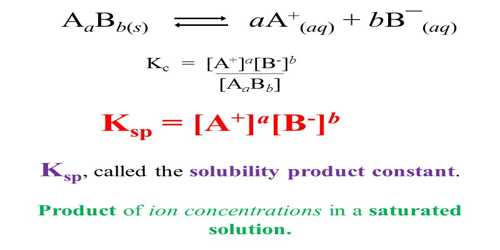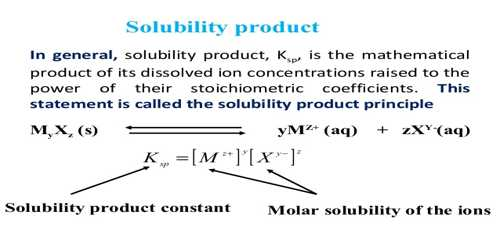Chemistry

# Calculation of Solubility Product from Solubility DataChemiCalculation of solubility product from solubility data

Solubility is defined as the maximum amount of solute that can be dissolved in a solvent at equilibrium. The solubility product constant (K) describes the equilibrium between a solid and its constituent ions in a solution. The solubility product of a compound can be calculated from solubility data. This will be explained with the help of few worked out examples.Example- 1: The solubility of silver chloride, AgCl, is 1.92 x 10-3 g L-1 at 25°C. Calculate the solubility product of AgCl at this temperature.

Solution:

In solution AgCl (s) ↔ Ag+ (aq) + Cl (aq)

Solubility in mol L-1 = [1.92 x 10-3 / 143.5] = 1.34 x 10-5

(Relative formula mass of AgCl = 143.51)

The concentration of each of the ions is equal to the solubility of the salt.

[Ag+] = [Cl] = 1.34 x 10-5

Ksp = [Ag+] = [Cl] = (1.34 x 10-5)2

= 1.8 x 10-10

Example- 2: At 250C the solubility of lead iodide, PbI2, is found to be 1.2 x 10-3 mol L-1. Calculate the solubility product, Ksp of lead iodide.

Solution

In solution, PbI2 (s) ↔ Pb2+ (aq) + 2I (aq).

The concentration, i.e. solubility, of the salt is equal to the concentration of Pb2+ ions. It can be seen that the concentration of iodide ion, I, is double that of lead ion, Pb2+.

[Pb2+] = 1.2 x 10-3 mol L-1.

[I] = 2 [Pb2+] = 2 x 1.2 x 10-3

= 2.4 x 10-3 mol L-1

Ksp = [Pb2+] [I]2 = (1.2 x 10-3) x (2.4 x 10-3)2

= 6.9 x 10-9.